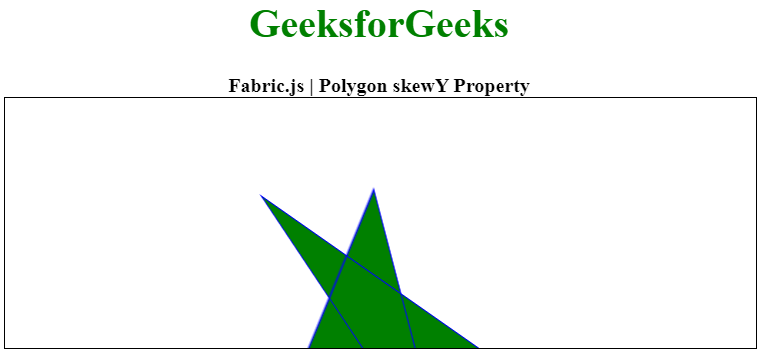Related Articles
Fabric.js Polygon skewY Property
• Last Updated : 20 Jan, 2021

In this article, we are going to see how to set the vertical skew of a canvas Polygon using FabricJS. The canvas Polygon means Polygon is movable and can be stretched according to requirement. Further, the Polygon can be customized when it comes to initial stroke color, height, width, fill color, or stroke width.

To make it possible, we are going to use a JavaScript library called FabricJS. After importing the library, we will create a canvas block in the body tag which will contain the Polygon. After this, we will initialize instances of Canvas and Polygon provided by FabricJS and set vertical skew of the Polygon using skewY property, and render the Polygon on the Canvas as given in the example below.

Syntax:

```fabric.Polygon([
{ x: pixel, y: pixel },
{ x: pixel, y: pixel },
{ x: pixel, y: pixel},
{ x: pixel, y: pixel},
{ x: pixel, y: pixel }
],
{
skewY : number
});```

Parameters: This property accepts a single parameter as mentioned above and described below:

• skewY: It specifies the vertical skew.

Below examples illustrate the Fabric.JS Polygon skewY in JavaScript:

Example:

## HTML

 ` ``<``html``> `` ` `<``head``>``    ````  ``<``script` `src``= ``"https://cdnjs.cloudflare.com/ajax/libs/fabric.js/3.6.2/fabric.min.js"``> ``  `` `` `` ` `<``body``> ``    ``<``div` `style``=``"text-align: center;width: 600px;"``> ``      ``<``h1` `style``=``"color: green;"``> ``          ``GeeksforGeeks ``      `` ``      ``<``b``> ``          ``Fabric.js | Polygon skewY Property ``      `` ``    ```` ` `    ``<``canvas` `id``=``"canvas"` `width``=``"600"` `height``=``"200"``        ``style``=``"border:1px solid #000000;"``> ``    `` `` ` `    ``<``script``> ``        ``// Initiate a Canvas instance ``        ``var canvas = new fabric.Canvas("canvas"); `` ` `        ``// Initiate a polygon instance ``        ``var polygon = new fabric.Polygon([ ``            ``{ x: 295, y: 10 }, ``            ``{ x: 235, y: 198 }, ``            ``{ x: 385, y: 78 }, ``            ``{ x: 205, y: 78 }, ``            ``{ x: 355, y: 198 }], {``             ``fill:'green',``             ``stroke: 'blue',``             ``paintFirst: 'fill',``             ``skewY: 35``        ``}); `` ` `        ``// Render the polygon in canvas ``        ``canvas.add(polygon); ``    `` `` `` ` ``

Output:My Personal Notes arrow_drop_up